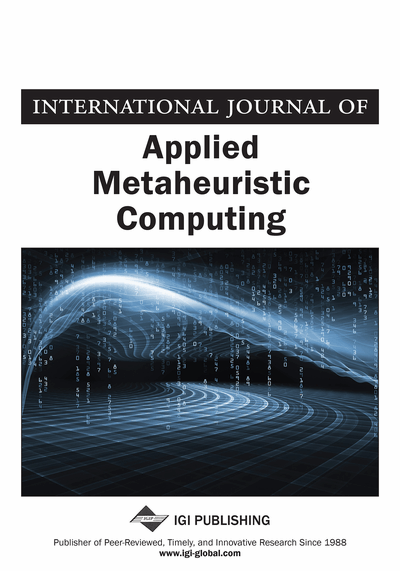# An Approximate Algorithm for Triangle TSP with a Four-Vertex-Three-Line Inequality

Yong Wang (School of Renewable Energy, North China Electric University, Beijing, China)
DOI: 10.4018/ijamc.2015010103

## Abstract

Traveling salesman problem (TSP) is a classic combinatorial optimization problem. The time complexity of the exact algorithms is generally an exponential function of the scale of TSP. This work gives an approximate algorithm with a four-vertex-three-line inequality for the triangle TSP. The time complexity is O(n2) and it can generate an approximation less than 2 times of the optimal solution. The paper designs a simple algorithm with the inequality. The algorithm is compared with the double-nearest neighbor algorithm. The experimental results illustrate the algorithm find the better approximations than the double-nearest neighbor algorithm for most TSP instances.
Article Preview
Top

## 1. Introduction

Given a tourist map with a set of n cities and the distances dij (1≤i,jn and ij) between each pairs of them, find the shortest tour which visits each of the cities once and exactly once. Here n is a finite integer. This is the classic traveling salesman problem (TSP). A tour with each of the cities once is named as the Hamiltonian cycle (HC) or Hamiltonian tour. The objective of TSP is to detect the shortest HC, i.e., the best tour with length lmin=Min(l(HC)) and where l(HC) means the length of the HC. In graph theory, the TSP map is usually represented as a complete weighted graph. The weights on the edges note the distance, time or expense for various kinds of TSP. Therefore, a mathematical formulation of TSP is to find the HC with the minimum (or maximum) weights in a complete weighted graph. In this paper, we take the minimum HC as the best tour and the weight is also called the cost.

TSP is easy to describe but hard to resolve. It has been proven to be NP-complete. For the symmetrical TSP with n cities, the number of HCs reaches (n-1)!/2. It is a challenging work to design an efficient algorithm for TSP. The time complexity of the exact algorithms is generally an exponential function of the scale of TSP. Finding the best tour is our desired goal whereas the exact algorithms are time-consuming for large scale of TSP instances. In 1962, Held and Karp (1962) and Bellman (1962) used the dynamic programming method to detect the best tour in O(n22n) time. Till 2010, the time is reduced to O(1.657n) by Andreas (Andreas, 2010) with a Monte Carlo method. Please refer to the literatures (Woeginger, 2003; Matai, Prakash & Mittal, 2010; Applegate, Bixby, Chvatal & Cook, 2006) to acquire more information about the exact algorithms for TSP. Although some TSP with thousands of cities has been resolved by the exact algorithms, such as the improved cutting plane method (http://www.math.uwaterloo.ca/tsp/index.html), it is believed the exact algorithm does not exist unless NP=P. The experiments on exact algorithms show that they are feasible for TSP with less than 1,000 cities (Singh, & Oudheusden, 1997; Klerk & Dobre, 2011; Gouveia, Voβ, 1995; Perez, & Gonzalez, 2004). Once the scale of TSP becomes larger, the computation time will increase tremendously even the super computers are utilized (Levine, 2000; Ergan & Orlin, 2006).

## Complete Article List

Search this Journal:
Reset
Open Access Articles: Forthcoming
Volume 11: 4 Issues (2020): 3 Released, 1 Forthcoming
Volume 10: 4 Issues (2019)
Volume 9: 4 Issues (2018)
Volume 8: 4 Issues (2017)
Volume 7: 4 Issues (2016)
Volume 6: 4 Issues (2015)
Volume 5: 4 Issues (2014)
Volume 4: 4 Issues (2013)
Volume 3: 4 Issues (2012)
Volume 2: 4 Issues (2011)
Volume 1: 4 Issues (2010)
View Complete Journal Contents Listing